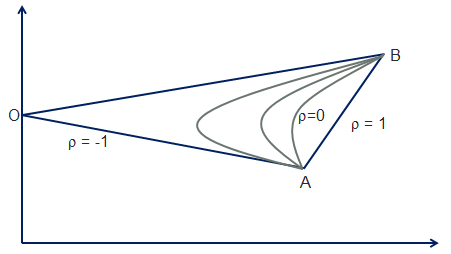# Effect of Correlation on Diversification

In this article, we will look at how correlation affects the diversification benefits of a portfolio. Let’s take a portfolio with two assets. The correlation between the two assets can range from -1.0 to 1.0 and depending on the correlation figure the shape of the efficient frontier will change.

The following graph shows how the efficient frontier will look based on different values of correlation. When the correlation between the assets is 1, it means that any change in one asset is equally reflected in the change in the other asset. So, if one asset goes down 10%, the other asset will also go down 10%. There is no diversification benefit. In such a case any portfolio derived from a combination of A and B will fall on the straight line, reflecting the risk simply weighted with both assets.However, as the correlation starts reducing, the portfolio starts getting diversification benefit and the efficient frontier starts bending towards the left. The lesser the correlation between the two assets, the more the diversification benefit and more it will bend towards the left. By this logic, when the two assets are perfectly negatively correlated, that is, the correlation between them is -1, the diversification should yield maximum risk reduction. So, there could be a combination of A and B where the risk reduces to 0. That point is represented as O in the above chart. It is not however really possible to find assets that are perfectly negatively correlated. For this reason the efficient frontier will usually be a curved line bending towards the left, signifying some levels of diversification.

Get our Data Science for Finance Bundle for just $29$51.